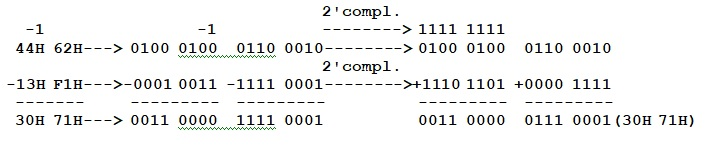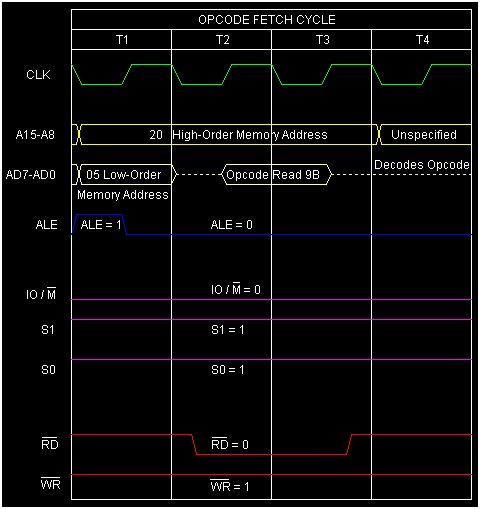# Instruction type SBB R in 8085 Microprocessor

In 8085 Instruction set, SBB R is mnemonic used for multi-Byte subtraction. Let us consider the following example on such subtractionIn this above example, the subtraction of 62H and F1H will result in 71H with a borrow of 1. Next, we have to subtract 44H and 13H along with this borrow value of 1. In the above tracing we have shown you how the internal calculations are being done. Now in 8085, to facilitate such an operation, SBB instruction has been provided to subtract two numbers along with the borrow value.

SBB is a mnemonic that stands for 'SuBtract with Borrow' and 'R' stands for any of the following 7 registers, and also memory location M as pointed by HL register pair.

R = A, B, C, D, E, H, L, or M


This instruction is used to compute subtraction between contents of R register from the Accumulator's content, along with the carry (borrow) value. The result of the subtraction will be stored in the Accumulator replacing Accumulator’s initial value. As R can have any of the eight values, there are eight opcodes for this type of instruction. It will occupy only 1-Byte in memory.

Mnemonics, Operand Opcode(in HEX) Bytes
SBB A 9F 1
SBB B 98 1
SBB C 99 1
SBB D 9A 1
SBB E 9B 1
SBB H 9C 1
SBB L 9D 1
SBB M 9E 1

Let us consider SBB E as an example instruction of this type. As it is a 1-Byte instruction so it will occupy a single memory location. Let us suppose, Accumulator’s initial content is 44H and E register’s initial content is 13H and Cy is set to 1. The result of execution of this instruction SBB E is shown below in the tracing table

Before After

(E)

13H 13H

(A)

44H 30H

(F)

Cy=1, other flag bits=any value Cy=0, AC=1,S=0,P=1,Z=0